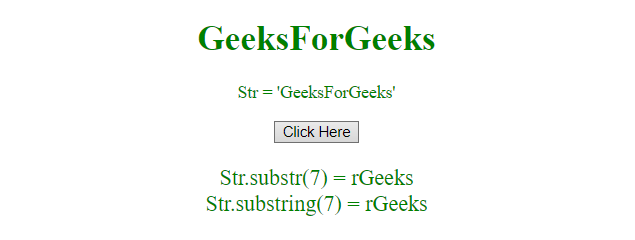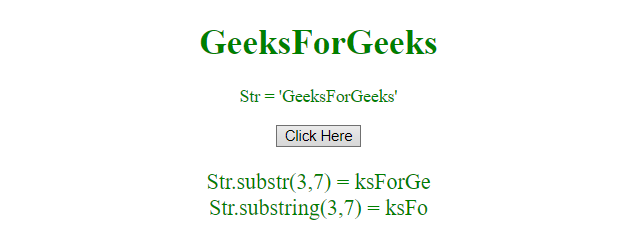# Difference between substr() and substring() in JavaScript

• Last Updated : 10 Apr, 2022

Both of the functions are used to get the specified part of the string, But there is a slight difference between them. str.substr() Function: The str.substr() function returns the specified number of characters from the specified index from the given string. Syntax:

`str.substr(start, len)`

Parameters:

• start: It is required parameter. It specifies the position from where to start the extraction. The index of the first character is 0. If the start parameter is positive and greater than, or equal, to the length of the str, then this function returns an empty string. If the start parameter is negative, then this function uses it as an index from the end of the string. If the start parameter is negative or greater than the length of string, the start parameter is set to 0.
• len: It is optional parameter. It specifies the number of characters to extract. If not passed, then it extracts the whole string.

str.substring() This method get the characters from a string, between two specified indices, and returns the new string. Syntax:

`str.substring(start, end)`

Parameters:

• start: It is required parameter. It specifies the position where to start the extraction. The index of first character is 0.
• end: It is optional parameter. It specifies the position (up to, but excluding) where to end the extraction. If not used, it extracts the whole string.

Example 1: This example uses only one parameter for both the functions and produces the same output.

## HTML

 ```<``html``>` `<``head``>``    ``<``title``>``        ``Difference between substr()``        ``and substring() function``    ````` `<``body` `style` `= ``"text-align:center;"``>``    ` `    ``<``h1` `style` `= ``"color:green;"` `>``        ``GeeksForGeeks``    ````    ` `    ``<``p` `id``=``"Geek_up"` `style``=``"color:green;"``>` `    ` `    ``<``button` `onclick` `= ``"Geeks()"``>``        ``Click Here``    ````    ` `    ``<``p` `id``=``"Geek_down"``        ``style``=``"color:green; font-size:20px;"``>` `    ` `    ``<``script``>``        ``var up = document.getElementById("Geek_up");``        ``var a = "GeeksForGeeks";``        ``up.innerHTML = "Str = " + "'" + a + "'";``        ` `        ``function Geeks() {``            ``var down = document.getElementById("Geek_down");``            ``down.innerHTML = "Str.substr(7) = " + a.substr(7) +``            ``"<``br``>" + "Str.substring(7) = " + a.substring(7);``        ``}``    ````` `                   `

Output:

• Before clicking on the button:• After clicking on the button:Example 2: This example uses the parameter (3, 7) for both functions and returns the output.

## html

 ```<``html``>` `<``head``>``    ``<``title``>``        ``JavaScript | Difference between``        ``substr() and substring() function``    ````` `<``body` `style` `= ``"text-align:center;"``>``    ` `    ``<``h1` `style` `= ``"color:green;"` `>``        ``GeeksForGeeks``    ````    ` `    ``<``p` `id``=``"Geek_up"` `style``=``"color:green;"``>` `    ` `    ``<``button` `onclick` `= ``"Geeks()"``>``        ``Click Here``    ````    ` `    ``<``p` `id``=``"Geek_down"``        ``style``=``"color:green; font-size:20px;"``>` `    ` `    ``<``script``>``        ``var up = document.getElementById("Geek_up");``        ``var a = "GeeksForGeeks";``        ``up.innerHTML = "Str = " + "'" + a + "'";``        ` `        ``function Geeks() {``            ``var down = document.getElementById("Geek_down");``            ``down.innerHTML = "Str.substr(3, 7) = " + a.substr(3, 7) +``            ``"<``br``>" + "Str.substring(3, 7) = " + a.substring(3, 7);``        ``}``    ````` `                   `

Output:

• Before clicking on the button:• After clicking on the button:Let us understand the differences in a tabular form -:

My Personal Notes arrow_drop_up